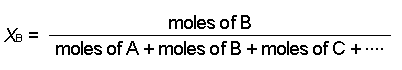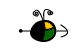Expressing Concentration

The concentration of a solution

• is a macroscopic property,
• represents the amount of solute dissolved in a unit amount of solvent or of solution, and
• can be expressed in a variety of ways (qualitatively and quantitatively).

Qualitative Expressions of Concentration

A solution can be qualitatively described as

• dilute: a solution that contains a small proportion of solute relative to solvent, or
• concentrated: a solution that contains a large proportion of solute relative to solvent.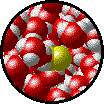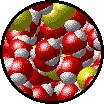Microscopic view of a dilute solution of liquid Br2 dissolved in liquid water. Microscopic view of a concentrated solution of liquid Br2 dissolved in liquid water.

Semi-Quantitative Expressions of Concentration

A solution can be semi-quantitatively described as

• unsaturated: a solution in which more solute will dissolve, or
• saturated: a solution in which no more solute will dissolve.

The solubility of a solute is the amount of solute that will dissolve in a given amount of solvent to produce a saturated solution. For example, at 0oC, we can dissolve a maximum of 35.7 g of solid NaCl in 100 mL of water (a saturated solution). Any additional solid NaCl that we add to the saturated solution simply falls to the bottom of the container and does not dissolve.

Quantitative Expressions of Concentration

There are a number of ways to express the relative amounts of solute and solvent in a solution. Which one we choose to use often depends on convenience. For example, it is sometimes easier to measure the volume of a solution rather than the mass of the solution.

Note that some expressions for concentration are temperature-dependent (i.e., the concentration of the solution changes as the temperature changes), whereas others are not. This is an important consideration for experiments in which the temperature does not remain constant.

Temperature Dependence of Several Concentration Expressions
concentration expression measurements required temperature dependent?
percent composition
(by mass)
mass of solute
mass of solution
no
(mass does not change with temperature)
molarity moles of solute
volume of solution
yes
(volume changes with temperature)
molality moles of solute
mass of solvent
no
(neither mass nor moles changes with temperature)
mole fraction moles of solute
moles of solvent
no
(moles does not change with temperature)

Percent Composition (by mass)

We can consider percent by mass (or weight percent, as it is sometimes called) in two ways:

• The parts of solute per 100 parts of solution.
• The fraction of a solute in a solution multiplied by 100.
We need two pieces of information to calculate the percent by mass of a solute in a solution:
• The mass of the solute in the solution.
• The mass of the solution.

Use the following equation to calculate percent by mass: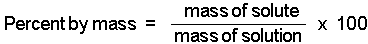Molarity

Molarity tells us the number of moles of solute in exactly one liter of a solution. (Note that molarity is spelled with an "r" and is represented by a capital M.)

We need two pieces of information to calculate the molarity of a solute in a solution:

• The moles of solute present in the solution.
• The volume of solution (in liters) containing the solute.

To calculate molarity we use the equation: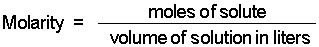Molality

Molality, m, tells us the number of moles of solute dissolved in exactly one kilogram of solvent. (Note that molality is spelled with two "l"'s and represented by a lower case m.)

We need two pieces of information to calculate the molality of a solute in a solution:

• The moles of solute present in the solution.
• The mass of solvent (in kilograms) in the solution.

To calculate molality we use the equation: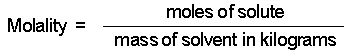Mole Fraction

The mole fraction, X, of a component in a solution is the ratio of the number of moles of that component to the total number of moles of all components in the solution.

To calculate mole fraction, we need to know:

• The number of moles of each component present in the solution.

The mole fraction of A, XA, in a solution consisting of A, B, C, ... is calculated using the equation: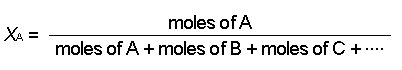To calculate the mole fraction of B, XB, use: